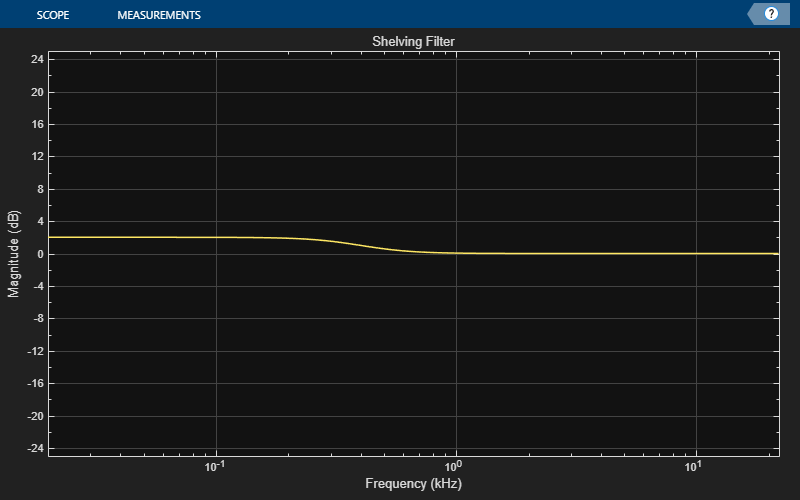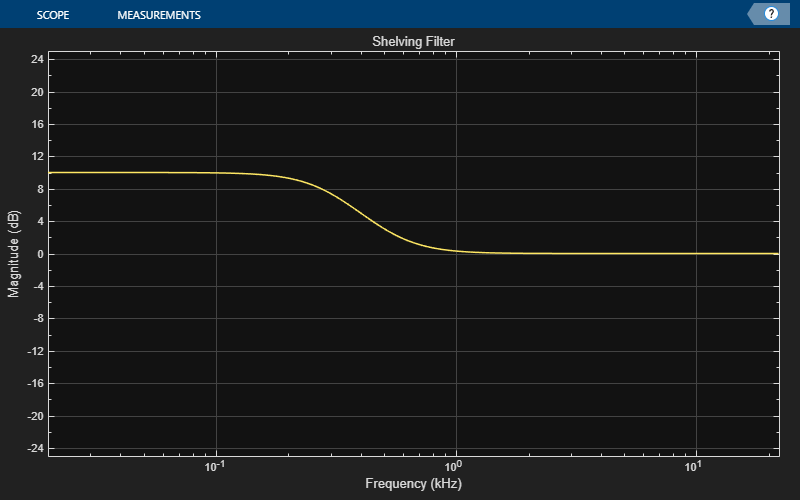# visualize

Visualize magnitude response of shelving filter

## Syntax

``visualize(shelvFilt)``
``visualize(shelvFilt,NFFT)``
``hvsz = visualize(___)``

## Description

example

````visualize(shelvFilt)` plots the magnitude response of the shelving filter. The plot is updated automatically when you change the object properties.```
````visualize(shelvFilt,NFFT)` specifies an N-point FFT to calculate the magnitude response.```
````hvsz = visualize(___)` returns a handle to the visualizer as a `dsp.DynamicFilterVisualizer` object when you call this syntax with any of the previous input arguments.```

## Examples

collapse all

Create a `shelvingFilter` object, and then call `visualize` to plot the magnitude response of the filter.

```shelvFilt = shelvingFilter(2,1,400,"lowpass"); visualize(shelvFilt)```Modify the gain and observe that the plot is updated automatically.

`shelvFilt.Gain = 10;`## Input Arguments

collapse all

Shelving filter whose magnitude response you want to plot, specified as a `shelvingFilter` System object™.

Number of bins used to calculate the DFT, specified as a positive scalar.

Data Types: `single` | `double` | `int8` | `int16` | `int32` | `int64` | `uint8` | `uint16` | `uint32` | `uint64`

## Version History

Introduced in R2022a